• 　所谓双边带调制（DSB）即载波抑制的调幅调制，它的载波被抑制，包络与调制前的信号不同，利用这一点即可实现信号的加密。加密时，将发话方语音信号调制在一个单音频信号上，从而产生一个经过DSB调制的音频信号（该...
• 做通信实验时的必备指导书，包括实验一 信号源实验 实验二 信道与眼图实验 实验四 常规双边带调幅与解调实验（AM）实验七 频分复用实验（FDM）等几个通信必做实验，相信能够对您的学习有所助益！
• 完整的，希望大家喜欢，通信原理课程设计，完整的。
• 文章目录1、模拟调制基本原理2、 1、模拟调制基本原理 问题的提出   考虑我们用麦克风采集语音信号，完成声-电转换，得到的电信号频率范围在20Hz~20kHz之间，显然属于低通信号。语音（电）信号为模拟随机信号，...
文章目录1、引言2、常规调幅调制(AM)调制器模型3、AM信号的傅立叶变换4、AM信号功率与调制效率5、抑制载波双边带(DSB-SC)调制
1、引言
考虑我们用麦克风采集语音信号，完成声-电转换，得到的电信号频率范围在20Hz~20kHz之间，显然属于低通信号。语音（电）信号为模拟随机信号，取值和时间上都是连续的，我们用$m(t)$表示。图1中，我们用录音机获取了周杰伦新歌《说好不哭》大约24s的音频，左边图为波形，右边为频率。不难看出，主要频率集中在零频到7kHz之间。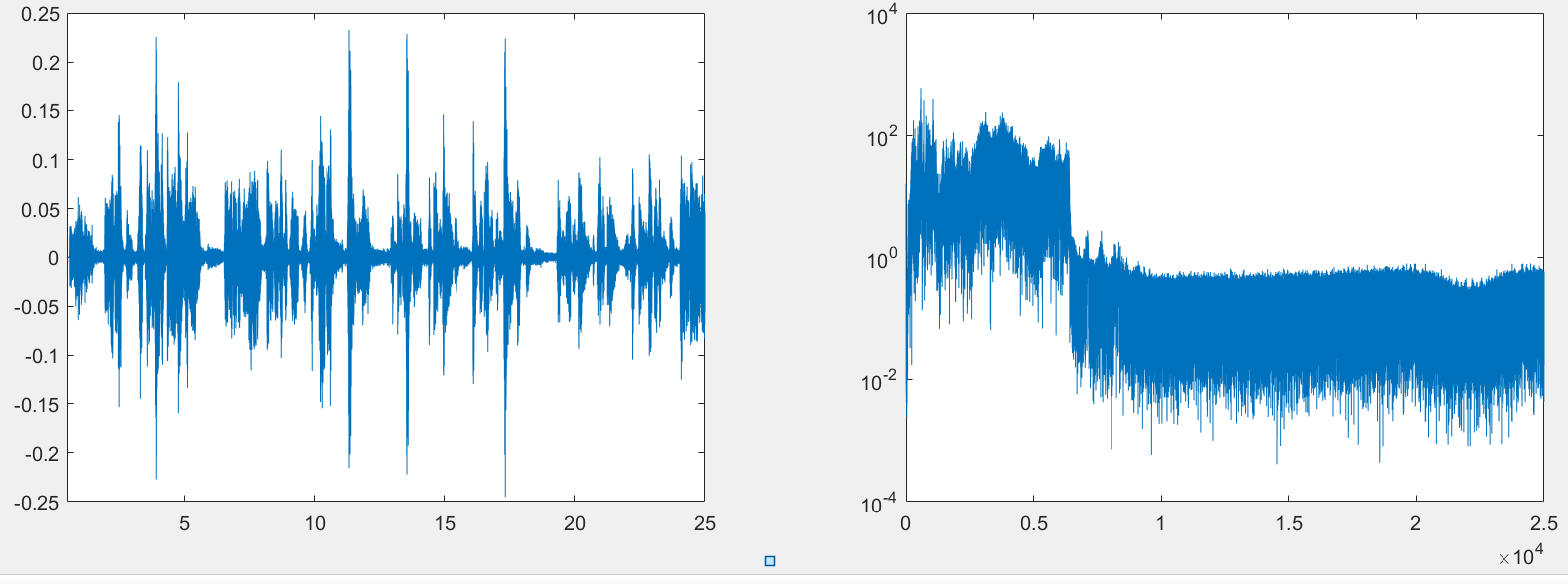图1 模拟基带信号
现在的问题是，如果我们想把类似图1中的这样的基带信号辐射到空间中，即采用无线方式进行传输，我们需要一定长度的天线。作为估算，一般来说我们认为天线长度需要等于无线电波长的$\frac{1}{4}$（当然真正做天线设计时需要更准确的计算），这就意味着，如果我们的基带信号频率为3kHz，波长$\lambda=\frac{c}{f}$，这里的$f=3$kHz为信号频率，$c=3\times 10^8$m/s为光速，因此天线长度大约需要2.5km，显然这是不可能的。如果我们把信号频率提升到300MHz，则天线长度只需要0.25m。
我们还可能遇到这样一个问题。在无线传输中，由于所有无线信号都在空中叠加，因此会产生严重干扰，因此需要通过无线电管理，为不同系统分配不同带宽。以4G移动通信系统为例，包括1880-1900MHz、2320-2370MHz、2575-2635MHz等。这也意味着，我们需要将低通语音或者数字信号的频率升高，变为带通信号，才能够在分配的频段内传输。当然，在接收端需要将信号从带通再进行下搬移，变回低通信号。而这种低通变带通，带通变低通的过程，就称为信号的调制与解调。
这一讲，主要介绍模拟幅度调制，包括常规调幅调制(AM)、抑制载波双边带(SSB-SC)调制以及单边带(SSB)调制。
2、常规调幅调制(AM)调制器模型
AM调制器模型如图1(a)所示。显然有
$\tag{1} s_{\rm AM}(t)=A_c[1+m(t)]\cos 2\pi f_ct.$进一步，我们定义调幅指数
$\tag{2} \beta_{\rm AM}=\max|m(t)|.$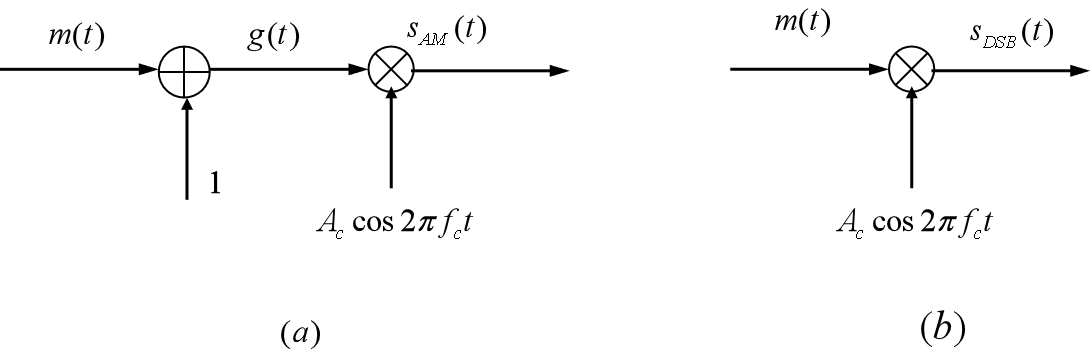图1 AM与DSB调制器模型
我们来看三种不同的调幅指数取值情况，即$\beta_{AM}<1$、$\beta_{AM}=1$以及$\beta_{AM}>1$的情况，如图2所示，其中(a)、(b)分别为基带信号和载波信号波形；(c )、(d)、(e)、(f)中调幅指数$\beta_{AM}$分别等于0.5、1、2和5。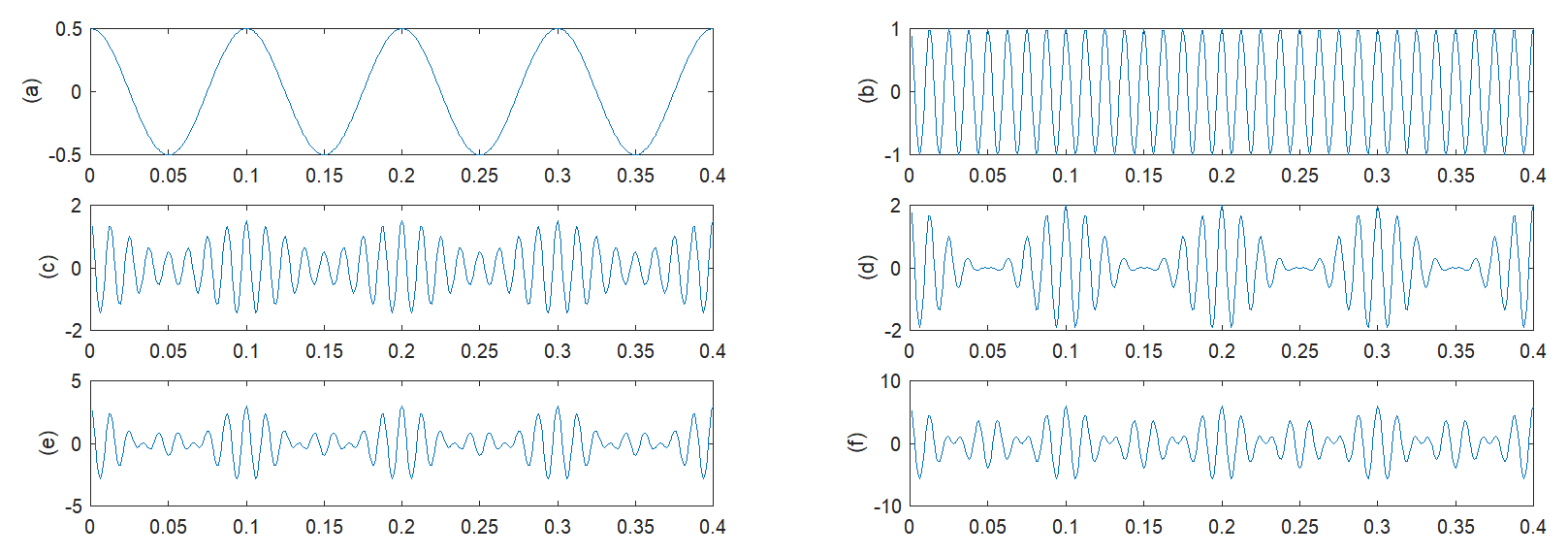图2 不同调幅指数时的AM系信号波形
事实上，AM调制的最大优点是接收机简单，可以采用包络检波法。这里我们不拟详述AM包络检波的详细过程，只在图3中给出示意，不难看出，二极管、电容和电阻即可构成包络检波器，通过电容充放电就可以跟随已调信号包络变化，而这里的包络，即(1)中的信号幅度$A_c[1+m(t)]$。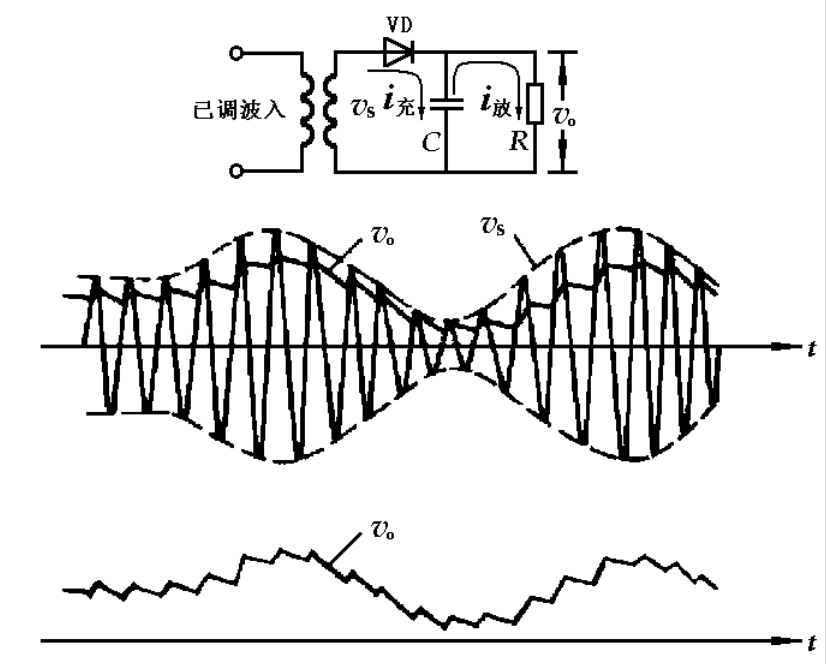图3 AM包络检波过程
当AM信号的调幅指数$\beta_{\rm AM}>1$时，包络检波会发生错误，如图4所示。此时，由于$1+m(t)$可能小于零，导致包络检波输出$|1+m(t)|$与之不等，故无法正确恢复出$m(t)$。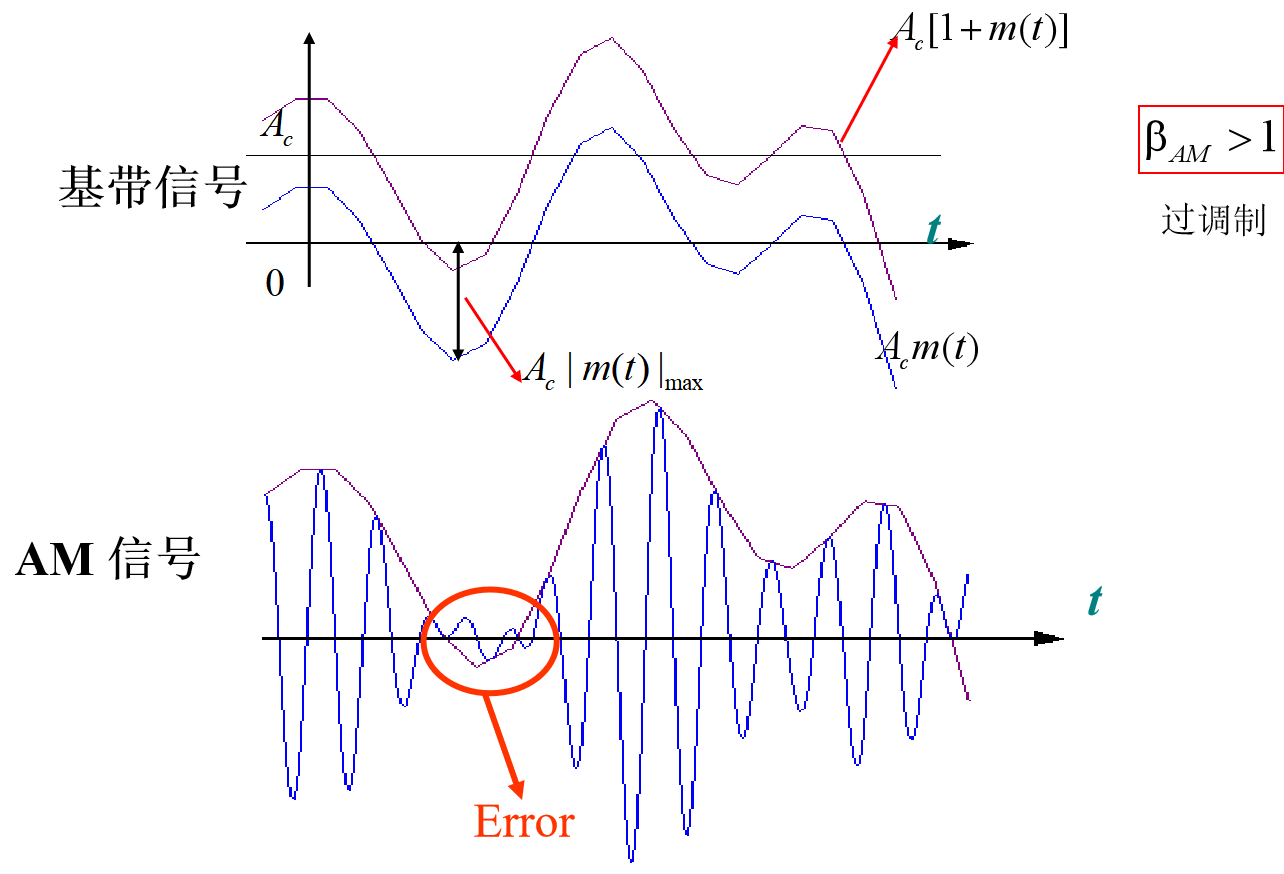图4 调幅指数大于1时包络检波出现错误
3、AM信号的傅立叶变换
根据(1)，我们可以得到AM信号的频谱密度为
$\tag{3} M(f)=\frac{A_c}{2}[\delta(f-f_c)+\delta(f+f_c)]+\frac{A_c}{2}[M(f-f_c)+M(f+f_c)].$其示意图如图5所示，这里$M(f)$为基带信号频谱，这里假定其最大频率（带宽）为$B$，显然AM信号包含两个部分，一是离散载波，在$f_c$处的冲激；二是边带信号，包括上边带（大于$f_c$)和下边带（小于$f_c$)。由于包含上下两个边带，已调的AM信号带宽为2B，因此我们称AM为双边带信号。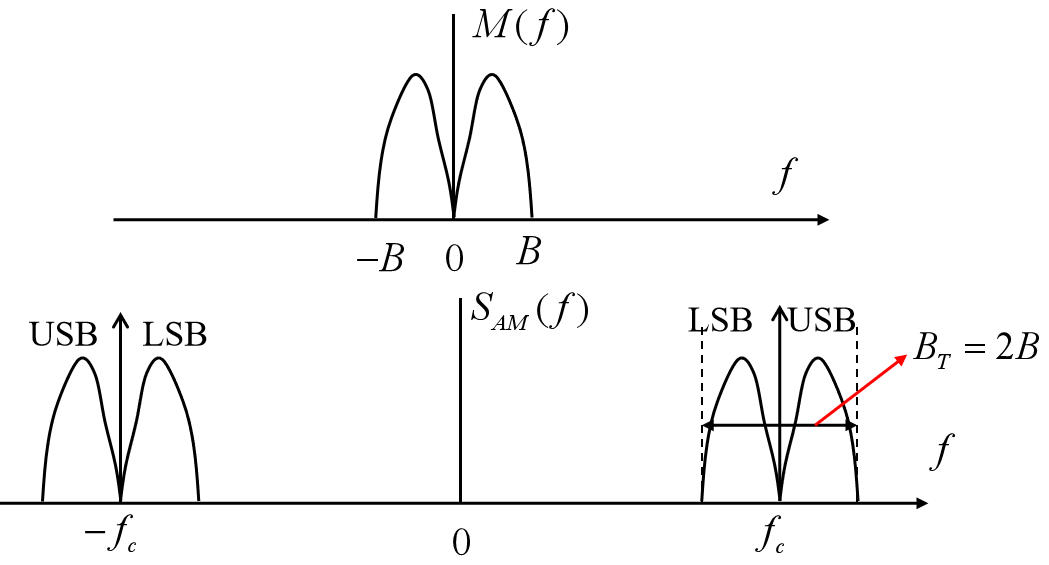图5 AM信号频谱密度示意图
4、AM信号功率与调制效率
下面我们来求AM信号的平均功率，有
\tag{4} \begin{aligned} \overline{s^2_{\rm AM}(t)}&=\overline{A_c^2[1+m(t)]^2\cos^2 2\pi f_ct}\\ &=\frac{1}{2}A_c^2\overline{[1+m(t)]^2}+\frac{1}{2}A_c^2\overline{[1+m(t)]^2\cos 4\pi f_ct},\\ \end{aligned}由于相对于$\cos 4\pi f_ct$，$m(t)$的变化要缓慢得多，因此可以近似认为在$\cos 4\pi f_ct$的一个周期之内，正负半周可以相互抵消，故上式中的第二项可以近似为0。进一步，我们有
\tag{4} \begin{aligned} \overline{s^2_{\rm AM}(t)}&=\frac{1}{2}A_c^2\overline{[1+m(t)]^2}\\ &=\frac{1}{2}A_c^2+\frac{1}{2}A_c^2\overline{m^2(t)}+A_c^2\overline{m(t)}, \end{aligned}我们一般假定$m(t)$为纯交流信号，即$\overline{m(t)}=0$，因此有AM信号的平均功率为
\tag{4} \begin{aligned} P_{\rm AM}&=P_c+P_m\\ &=\frac{1}{2}A_c^2+\frac{1}{2}A_c^2\overline{m^2(t)}, \end{aligned}这里，$P_c=\frac{1}{2}A_c^2$以及$P_m=\frac{1}{2}A_c^2\overline{m^2(t)}$分别称为载波功率和边带功率。显然，只有边带功率是用来传输基带信号$m(t)$的，据此，我们定义AM信号的调制效率为
\tag{4} \begin{aligned} \eta_{\rm AM}&=\frac{P_m}{P_c+P_m}=\frac{\frac{1}{2}A_c^2\overline{m^2(t)}}{\frac{1}{2}A_c^2+\frac{1}{2}A_c^2\overline{m^2(t)}}\\ &=\frac{\overline{m^2(t)}}{1+\overline{m^2(t)}}. \end{aligned}

【思考题1】请问$m(t)$为什么波形时调制效率最高？此时调制效率为多少？
【思考题2】如果$m(t)=a\cos 2\pi f_mt$为单音信号，则$a$等于多少是可以得到最大的调制效率？此最大调制效率等于多少？

5、抑制载波双边带(DSB-SC)调制
抑制载波双边带调制(double sideband suppressed carrier, DSB-SC)调制器如图1(b)所示。显然，与AM不同之处在于，DSB-SC直接用$m(t)$进行调制，其已调信号的时域与频域表达式分别为
\begin{aligned} s_{\rm DSB}(t)&=m(t)\cos 2\pi f_ct,\\ S_{\rm DSB}(f)&=\frac{A_c}{2}[M(f-f_c)+M(f+f_c)]. \end{aligned}DSB-SC信号的傅立叶变换与AM相比，没有载频处的冲激，只有边带成分。显然，DSB-SC信号的平均功率为
\tag{4} \begin{aligned} P_{\rm DSB}&=\frac{1}{2}A_c^2\overline{m^2(t)}. \end{aligned}


展开全文•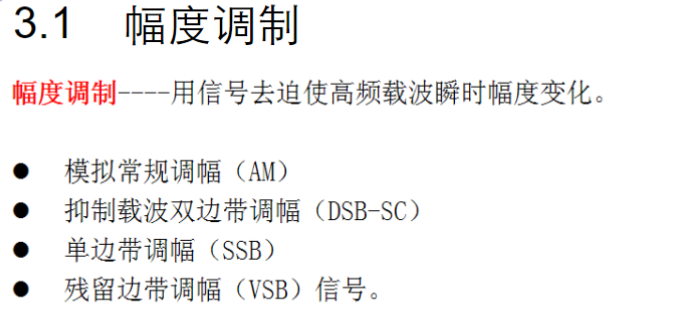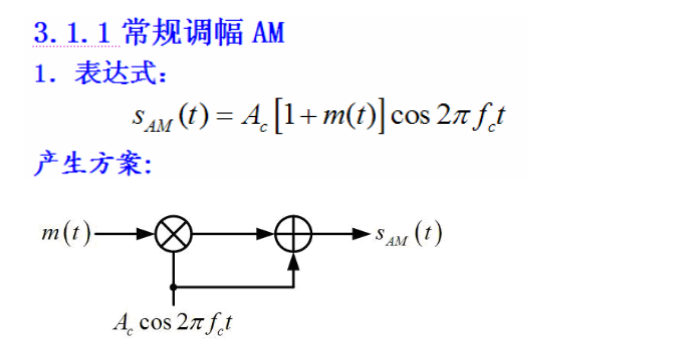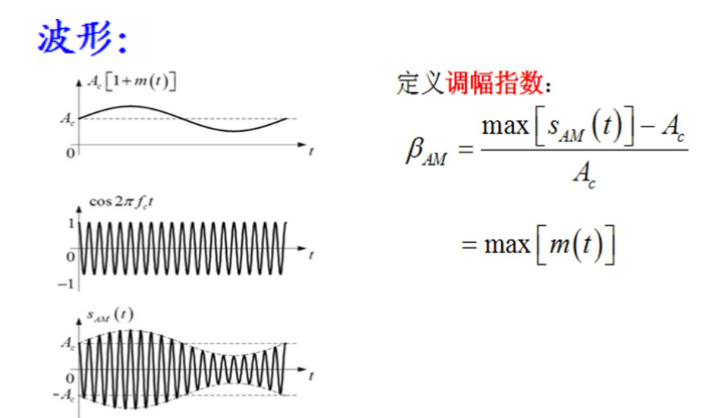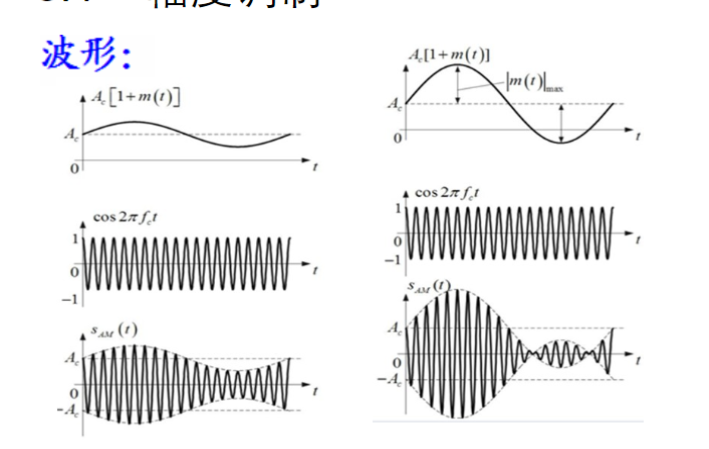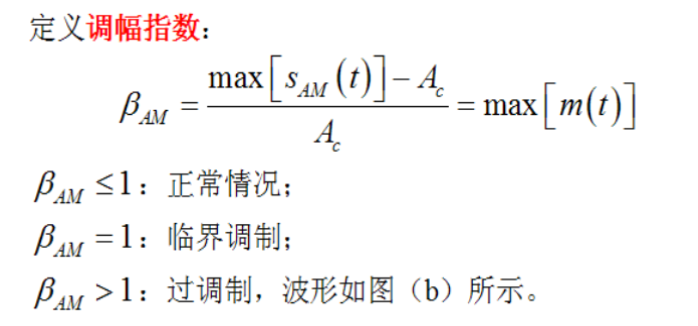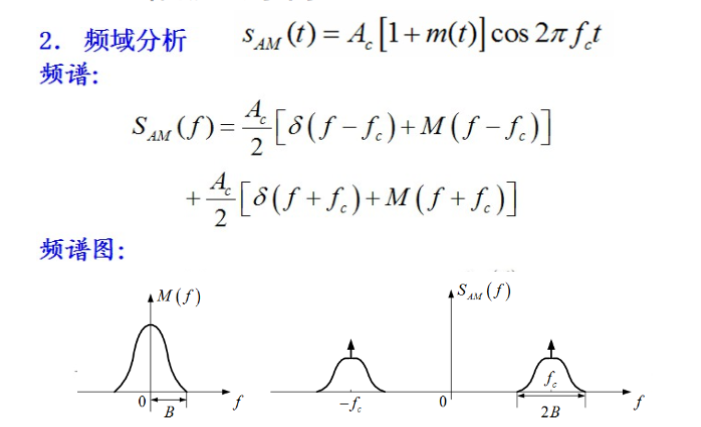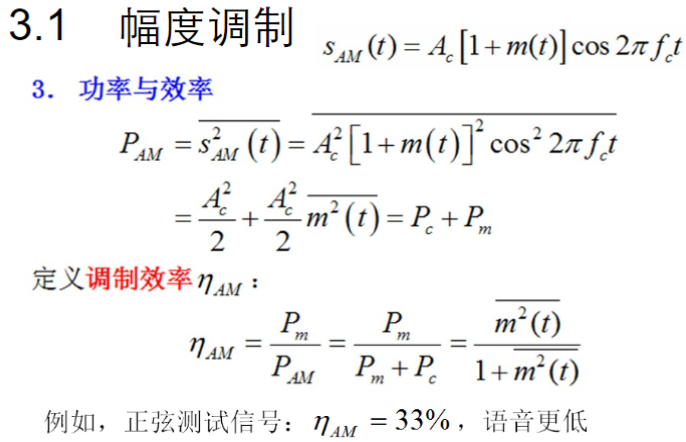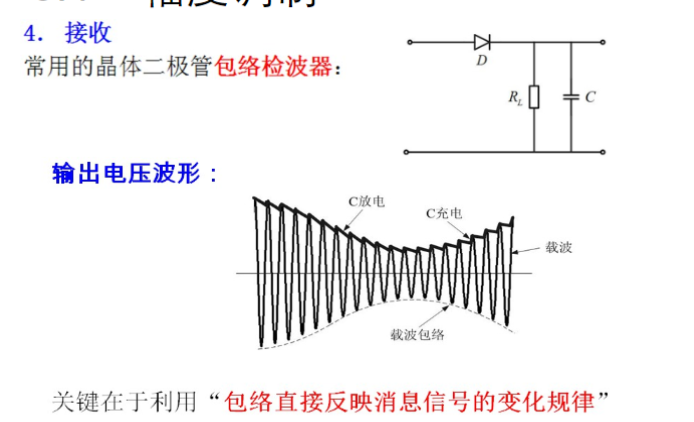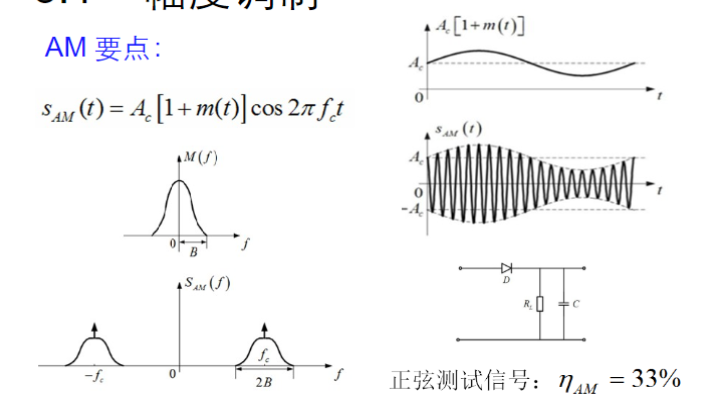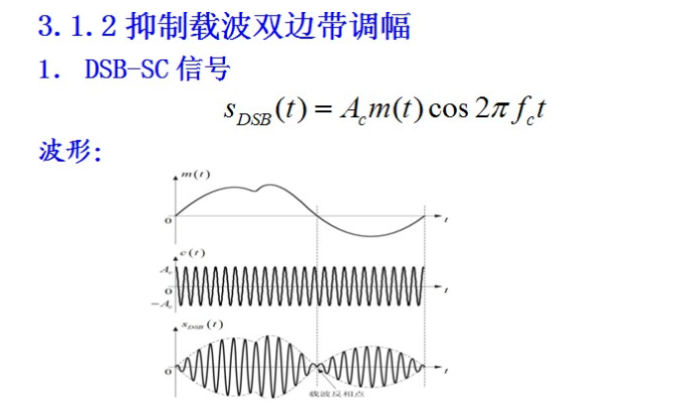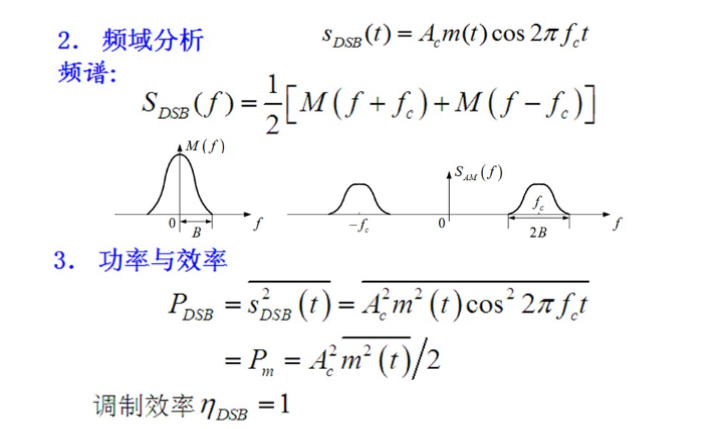展开全文• 此matlab代码，给出了现代通信原理中的常规双边带调幅(AM)的matlab仿真程序，给出了调制与解调的过程，并分析了在不同信噪比条件下的解调性能。
• c(t)=cos⁡(200πt)\color{Brown}{c(t) = \cos(200 \pi t)}c(t)=cos(200πt), 频率 fc=100Hzf_c = 100Hzfc​=100Hz 图表3 表示调制信号 s(t)=m(t)c(t)=cos⁡(20πt)cos⁡(200πt)\color{Brown}{s(t) = m(t) c(t) = \...

0. 作业要求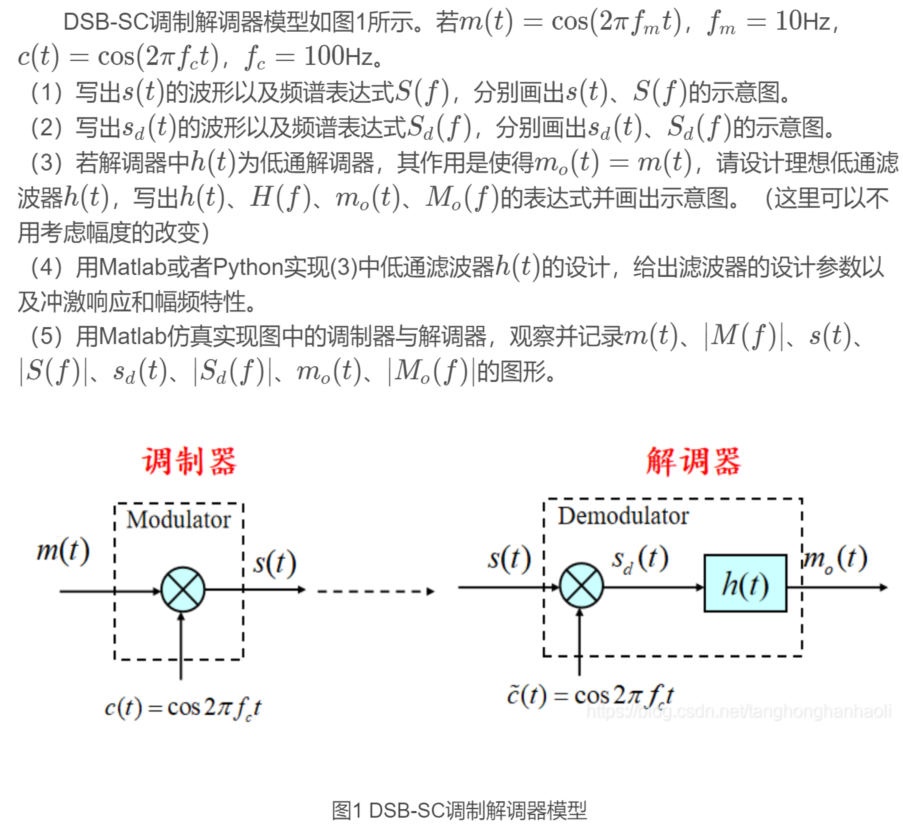1. $\color{Brown}{m(t), c(t), s(t)}$ 与 $\color{Brown}{M(f), C(f), S(f)}$ 的表达式与波形图
1.1 表达式
\begin{alignedat}{2} s(t) & = m(t) c(t) = \cos(20 \pi t)\cos(200 \pi t) \\ S(f) & = \frac{1}{4} \bigg [\delta(f - 110) + \delta(f - 90) + \delta(f + 90) + \delta(f + 110) \bigg ] \end{alignedat}
1.2 波形图

$\color{Brown}{m(t), c(t), s(t)}$ - 时域波形图表1 表示基带信号 $\color{Brown}{m(t) = \cos(20 \pi t)}$, 频率 $f_m = 10Hz$
图表2 表示载波信号 $\color{Brown}{c(t) = \cos(200 \pi t)}$, 频率 $f_c = 100Hz$
图表3 表示调制信号 $\color{Brown}{s(t) = m(t) c(t) = \cos(20 \pi t)\cos(200 \pi t)}$

$\color{Brown}{M(f)}$ - 频域波形图表1 表示信号 m(t) 的幅度频谱 |M(f)|
图表2 表示信号 m(t) 的相位频谱 θᴍ(f)
图表3 表示信号 m(t) 频率区间为 0~250Hz 的幅度频谱 |M(f)|
图表4 表示信号 m(t) 频率区间为 0~250Hz 的相位频谱 θᴍ(f)

$\color{Brown}{C(f)}$ - 频域波形图表1 表示信号 c(t) 的幅度频谱 |C(f)|
图表2 表示信号 c(t) 的相位频谱 θᴄ(f)
图表3 表示信号 c(t) 频率区间为 0~250Hz 的幅度频谱 |C(f)|
图表4 表示信号 c(t) 频率区间为 0~250Hz 的相位频谱 θᴄ(f)

$\color{Brown}{S(f)}$ - 频域波形图表1 表示信号 s(t) 的幅度频谱 |S(f)|
图表2 表示信号 s(t) 的相位频谱 θs(f)
图表3 表示信号 s(t) 频率区间为 0~250Hz 的幅度频谱 |S(f)|
图表4 表示信号 s(t) 频率区间为 0~250Hz 的相位频谱 θs(f)

2. $\color{Brown}{s_d(t)}$ 与 $\color{Brown}{S_d(f)}$ 的表达式与波形图
2.1 表达式
\begin{alignedat}{2} & \begin{aligned} s_d(t) & = s(t) \tilde{c}(t) \\ & = s(t) \cos(200 \pi t) \\ & = m(t) \cos^2(200 \pi t) \\ & = m(t) \frac{\cos(400 \pi t + 1)}{2} \\ & = \frac{1}{2} m(t) + \frac{1}{2} m(t) \cos(400 \pi t) \\ & = \frac{1}{2} \cos(20 \pi t) + \frac{1}{2} \cos(20 \pi t) \cos(400 \pi t) \\ \end{aligned} \\ & S_d(f) = \frac{1}{4} \bigg [\delta(f - 10) + \delta(f + 10) \bigg ] + \frac{1}{8} \bigg [\delta(f - 210) + \delta(f - 190) + \delta(f + 190) + \delta(f + 210) \bigg ] \end{alignedat}
2.2 波形图

$\color{Brown}{s(t) \ \tilde{c}(t) \ s_d(t)}$ - 时域波形图表1 表示调制信号 $\color{Brown}{s(t) = m(t) c(t) = \cos(20 \pi t)\cos(200 \pi t)}$
图表2 表示移频信号 $\color{Brown}{\tilde{c}(t) = \cos(200 \pi t)}$, 频率 $f_c = 100Hz$
图表2 表示移频后的调制信号 $\color{Brown}{s_d(t) = \frac{1}{2} \cos(20 \pi t) + \frac{1}{2} \cos(20 \pi t) \cos(400 \pi t)}$

$\color{Brown}{S_d(f)}$ - 频域波形图表1 表示信号 sᴅ(t) 的幅度频谱 |Sᴅ(f)|
图表2 表示信号 sᴅ(t) 的相位频谱 θs(f)
图表3 表示信号 sᴅ(t) 频率区间为 0~100Hz 的幅度频谱 |Sᴅ(f)|
图表4 表示信号 sᴅ(t) 频率区间为 0~100Hz 的相位频谱 θsᴅ(f)

3. $\color{Brown}{h(t)}$, $\color{Brown}{H(f)}$ 与 $\color{Brown}{m_o(t)}$, $\color{Brown}{M_o(f)}$ 的表达式与波形图
3.1 表达式
\begin{alignedat} \ h(t) = 4f_HSa(2 \pi f_H t) & \overset{\mathcal{F}}{\longleftrightarrow} H(f) = 2Rect(\frac{f}{2f_H}) \\ m_o(t) = s_d(t) * h(t) = \cos(20 \pi t) & \overset{\mathcal{F}}{\longleftrightarrow} M_o(f) = S_d(t)H(f) = \frac{1}{2} \bigg [\delta(f - 10) + \delta(f + 10) \bigg ] \\ \end{alignedat}
3.2 波形图

$\color{Brown}{h(t)}$ - 时域波形图表1 表示抽样函数 $\color{Brown}{h(t) = 4f_HSa(2 \pi f_H t)}$

$\color{Brown}{H(f)}$ - 频域波形图表1 表示抽样函数 h(t) 的幅度频谱 |H(f)|
图表2 表示抽样函数 h(t) 的相位频谱 θʜ(f)
图表3 表示抽样函数 h(t) 频率区间为 0~250Hz 的幅度频谱 |H(f)|
图表4 表示抽样函数 h(t) 频率区间为 0~250Hz 的相位频谱 θʜ(f)

$\color{Brown}{m_o(t)}$ - 时域波形图表1 表示移频滤波解调后的调制信号 $\color{Brown}{m_o(t) = s_d(t) * h(t)}$
图表2 表示原基带信号 $\color{Brown}{m(t) = \cos(20 \pi t)}$
图表2 表示去除滤波器产生的延迟后的解调信号 $\color{Brown}{m_o(t) = \cos(20 \pi t)}$

$\color{Brown}{M_o(f)}$ - 频域波形图表1 表示信号 mᴏ(t) 的幅度频谱 |Mᴏ(f)|
图表2 表示信号 mᴏ(t) 的相位频谱 θᴍ(f)
图表3 表示信号 mᴏ(t) 频率区间为 0~250Hz 的幅度频谱 |Mᴏ(f)|
图表4 表示信号 mᴏ(t) 频率区间为 0~250Hz 的相位频谱 θᴍ(f)

4. 其他
4.1 参考文章

 MATLAB通信仿真实例1：无噪声信道下DSB-SC调制解调器

4.2 仿真代码
# DSB-SC.py
# %% 配置导入
from scipy import signal
from scipy import fft
import scipy.constants as const
import matplotlib.pyplot as plt
import numpy as np

# %% 参数设置
time_begin = 0  # 起始时间
time_end = 1  # 终止时间
points = 1001  # 栅网采样点数
T = (time_end - time_begin) / (points - 1)  # 栅网采样间距
frequency = [10, 100]  # 频率
amplitude = [1, 1]  # 振幅
phase = [0, 0]  # 相位(cos(t)相位为0)
zoom = 4  # 频谱缩放倍数
tick_max = max(amplitude) * 1.5  # 波形纵轴刻度最小值
tick_min = -tick_max  # 波形纵轴刻度最大值

# %% 生成时域波形
t = np.linspace(time_begin, time_end, points)  # 生成时域栅网
waves = []
for i in range(len(frequency)):
waves.append(
signal.chirp(
t,
f0=frequency[i],
t1=time_end,
f1=frequency[i],
phi=phase[i],
method='linear'
) * amplitude[i]
)
s = None
for wave in waves:
if s is None:
s = wave.copy()
else:
s *= wave
s_d = waves * waves * waves

# %% 获得频域波形
f = np.linspace(0, 1 / T, points)  # 生成频域栅网
# 输入信号 M(f)
M = fft.fft(waves)  # 快速傅里叶变换
M_nor = M / points  # 归一化
M_mod = np.abs(M_nor)  # 获取幅度频谱
M_pha = np.angle(M)  # 获取相位频谱
# 移频信号 C(f)
C = fft.fft(waves)  # 快速傅里叶变换
C_nor = C / points  # 归一化
C_mod = np.abs(C_nor)  # 获取幅度频谱
C_pha = np.angle(C)  # 获取相位频谱
# 第一次移频 -> S(f)
S = fft.fft(s)  # 快速傅里叶变换
S_nor = S / points  # 归一化
S_mod = np.abs(S_nor)  # 获取幅度频谱
S_pha = np.angle(S)  # 获取相位频谱
# 第二次移频 -> S_d(f)
S_d = fft.fft(s_d)
S_d_nor = S_d / points  # 归一化
S_d_mod = np.abs(S_d_nor)  # 获取幅度频谱
S_d_pha = np.angle(S_d)  # 获取相位频谱

# %% 滤波器参数
f_H = 20  # 上限截止频率
time_begin_h = 0
time_end_h = 0.5
points_h = 501
T_h = (time_end_h - time_begin_h) / (points_h - 1)
frequency_h = f_H
amplitude = 4 * f_H
phase_h = -90
zoom_h = 10
tick_max_h = 4 * f_H
tick_min_h = -tick_max_h

# %% 生成抽样信号
t_h = np.linspace(time_begin_h, time_end_h, points_h)
h = amplitude * signal.chirp(
t_h,
f0=frequency_h,
t1=time_end_h,
f1=frequency_h,
phi=phase_h,
method='linear'
)  # 获得正弦函数
# 获得半个抽样信号
h = 4 * f_H
h[1:] /= (2 * const.pi * f_H * t_h[1:])
# 对称延拓抽样信号
t_h = np.hstack((-t_h[-1:0:-1], t_h))
h = np.hstack((h[-1:0:-1], h))

# %% 获得滤波器频域波形
f_h = np.linspace(0, 1 / T, points)  # 生成频域栅网
H = fft.fft(h)  # 快速傅里叶变换
H_nor = H / points  # 归一化
H_mod = np.abs(H_nor)  # 获取幅度频谱
H_pha = np.angle(H)  # 获取相位频谱

# %% 进行滤波, 获得 m_o(t) 的时域波形
m_o_original = signal.convolve(s_d, h) / points  # s_d(t) 与 h(t) 卷积
t_m_original = np.linspace(
time_begin, T * (len(m_o_original) - 1), len(m_o_original))  # m_o(t) 信号的栅网
m_o = m_o_original[points//2:points + points//2]  # 将通过滤波器之后产生的延迟截断

# %% 获得 m_o(t) 的频域波形
M_o = fft.fft(m_o)
M_o_nor = M_o / points  # 归一化
M_o_mod = np.abs(M_o_nor)  # 获取幅度频谱
M_o_pha = np.angle(M_o)  # 获取相位频谱

# %% 波形绘制函数
def drawWave(
figure_i,
row_i,
column_j,
label_x,
axis_xs,
axis_ys,
formats,
labels,
label_locs,
limit_ys,
linewidths):
"""
param:
row_i: 图表的行数
column_j: 图表的列数
label_x: 横轴标签
axis_xs: 横轴
axis_ys: 纵轴
formats: 曲线格式(颜色 风格 标记)
颜色:
'b' 蓝色(blue)(默认)
'g' 绿色(green)
'r' 红色(red)
'y' 黄色(yellow)
'k' 黑色(black)
'w' 白色(white)
'c' 青绿色(cyan)
'm' 洋红色(magenta)
'#xxxxxx'   RGB颜色
'0.xxx' 灰度
风格:
'-' 实线(默认)
':' 虚线
' ' 无线条
'--'    破折线
'-.'    点划线
标记:
'.' 点(默认)
',' 极小点
'o' 实心圆
'^' 正三角
'v' 倒三角
'>' 右三角
'<' 左三角
'1' 下花三角
'2' 上花三角
'3' 左花三角
'4' 右花三角
's' 实心方形(square)
'p' 实心五边形(pentagon)
'*' 星形
'h' 竖六边形(hexagon)
'H' 横六边形
'+' 十字
'x' 叉形
'D' 菱形(diamond)
'd' 瘦菱形
'|' 垂直线
labels: 曲线标签内容
label_locs: 曲线标签位置
0 "best 最佳位置(默认)
1 "upper right" 右上
2 "upper left" 左上
3 "lower right" 右下
4 "lower left" 左下
limit_ys: 坐标轴下限与上限
linewidths: 线宽
"""

fig = plt.figure(figure_i)
chart_formate = row_i * 100 + column_j * 10
for i in range(row_i * column_j):
ax = fig.add_subplot(chart_formate + 1 + i)
ax.plot(
axis_xs[i],
axis_ys[i],
formats[i],
label=labels[i],
linewidth=linewidths[i]
)  # 横轴, 纵轴, 标签
ax.legend(loc=label_locs[i])  # 标签位置
if limit_ys[i] is not None:
ax.set_ylim(limit_ys[i])  # 纵轴刻度范围
ax.grid()  # 显示网格线
ax.set_xlabel(label_x)

# %% 绘制 m(t) c(t) s(t) 时域波形
drawWave(
figure_i=1,
row_i=3,
column_j=1,
label_x=r"t / s",
axis_xs=[t] * 3,
axis_ys=waves + [s],
formats=['b-,', 'g-,', 'r-,'],
labels=[r"m(t) = cos(20πt)",
r"c(t) = cos(200πt)",
r"s(t) = m(t)c(t)"],
label_locs=[r"upper right"] * 3,
limit_ys=[(tick_min, tick_max)] * 3,
linewidths= * 3
)

# %% 绘制 m(t) 频域波形
drawWave(
figure_i=2,
row_i=4,
column_j=1,
label_x=r"f / Hz",
axis_xs=[np.hstack((-f[-1:0:-1], f))] * 2 + [f[0:points//zoom]] * 2,
axis_ys=[np.hstack((M_mod[-1:0:-1], M_mod)),
np.hstack((-M_pha[-1:0:-1], M_pha)),
M_mod[0: points // zoom],
M_pha[0: points // zoom]],
formats=['b-,', 'g-,'] * 2,
labels=[r"|M(f)|", r"θᴍ(f)"] * 2,
label_locs=[r"upper right"] * 4,
limit_ys=[(0, 0.6), None] * 2,
linewidths= * 4
)

# %% 绘制 c(t) 频域波形
drawWave(
figure_i=3,
row_i=4,
column_j=1,
label_x=r"f / Hz",
axis_xs=[np.hstack((-f[-1:0:-1], f))] * 2 + [f[0:points//zoom]] * 2,
axis_ys=[np.hstack((C_mod[-1:0:-1], C_mod)),
np.hstack((-C_pha[-1:0:-1], C_pha)),
C_mod[0: points // zoom],
C_pha[0: points // zoom]],
formats=['b-,', 'g-,'] * 2,
labels=[r"|C(f)|", r"θᴄ(f)"] * 2,
label_locs=[r"upper right"] * 4,
limit_ys=[(0, 0.6), None] * 2,
linewidths= * 4
)

# %% 绘制 s(t) 频域波形
drawWave(
figure_i=4,
row_i=4,
column_j=1,
label_x=r"f / Hz",
axis_xs=[np.hstack((-f[-1:0:-1], f))] * 2 + [f[0:points//zoom]] * 2,
axis_ys=[np.hstack((S_mod[-1:0:-1], S_mod)),
np.hstack((-S_pha[-1:0:-1], S_pha)),
S_mod[0: points // zoom],
S_pha[0: points // zoom]],
formats=['b-,', 'g-,'] * 2,
labels=[r"|S(f)|", r"θs(f)"] * 2,
label_locs=[r"upper right"] * 4,
limit_ys=[(0, 0.3), None] * 2,
linewidths= * 4
)

# %% 绘制 s(t) c(t) s_d(t) 时域波形
drawWave(
figure_i=5,
row_i=3,
column_j=1,
label_x=r"t / s",
axis_xs=[t] * 3,
axis_ys=[s, waves, s_d],
formats=['b-,', 'g-,', 'r-,'],
labels=[r"s(t) = m(t)c(t)",
r"c(t) = cos(200πt)",
r"s_d(t) = s(t)c(t)"],
label_locs=[r"upper right"] * 3,
limit_ys=[(tick_min, tick_max)] * 3,
linewidths= * 3
)

# %% 绘制 s_d(t) 频域波形
drawWave(
figure_i=6,
row_i=4,
column_j=1,
label_x=r"f / Hz",
axis_xs=[np.hstack((-f[-1:0:-1], f))] * 2 + [f[0:points//zoom]] * 2,
axis_ys=[np.hstack((S_d_mod[-1:0:-1], S_d_mod)),
np.hstack((-S_d_pha[-1:0:-1], S_d_pha)),
S_d_mod[0: points // zoom],
S_d_pha[0: points // zoom]],
formats=['b-,', 'g-,'] * 2,
labels=[r"|Sᴅ(f)|", r"θsᴅ(f)"] * 2,
label_locs=[r"upper right"] * 4,
limit_ys=[(0, 0.3), None] * 2,
linewidths= * 4
)

# %% 绘制 h(t) 时域波形
drawWave(
figure_i=7,
row_i=1,
column_j=1,
label_x=r"t / s",
axis_xs=[t_h],
axis_ys=[h],
formats=['b-,'],
labels=[r"h(t) = 2fʜSa(2πfʜt)"],
label_locs=[r"upper right"],
limit_ys=[(-5*f_H, 5*f_H)],
linewidths=
)

# %% 绘制 h(t) 频域波形
drawWave(
figure_i=8,
row_i=4,
column_j=1,
label_x=r"f / Hz",
axis_xs=[np.hstack((-f_h[-1:0:-1], f_h))] * 2 +
[f_h[0:points//zoom_h]] * 2,
axis_ys=[np.hstack((H_mod[-1:0:-1], H_mod)),
np.hstack((-H_pha[-1:0:-1], H_pha)),
H_mod[0: points // zoom_h],
H_pha[0: points // zoom_h]],
# axis_xs=[f_h] * 2,
# axis_ys=[H_mod,
#          H_pha],
formats=['b-,', 'g-,'] * 2,
labels=[r"|H(f)|", r"θʜ(f)"] * 2,
label_locs=[r"upper right"] * 4,
limit_ys=[(0, 2.5), None] * 2,
linewidths= * 4
)

# %% 绘制 m_o(t) 时域波形
drawWave(
figure_i=9,
row_i=3,
column_j=1,
label_x=r"t / s",
axis_xs=[t_m_original, t, t],
axis_ys=[m_o_original, waves, m_o],
formats=['b-,', 'g-,', 'r-,'],
labels=[r"m_o(t) = s_d(t) * h(t)", r"m(t)", r"m_o(t)"],
label_locs=[r"upper right"] * 3,
limit_ys=[(-1.5, 1.5)] * 3,
linewidths= * 3
)

# %% 绘制 m_o(t) 频域波形
drawWave(
figure_i=10,
row_i=4,
column_j=1,
label_x=r"f / Hz",
axis_xs=[np.hstack((-f[-1:0:-1], f))] * 2 +
[f[0:points//zoom]] * 2,
axis_ys=[np.hstack((M_o_mod[-1:0:-1], M_o_mod)),
np.hstack((-M_o_pha[-1:0:-1], M_o_pha)),
M_o_mod[0: points // zoom],
M_o_pha[0: points // zoom]],
# axis_xs=[f_h] * 2,
# axis_ys=[H_mod,
#          H_pha],
formats=['b-,', 'g-,'] * 2,
labels=[r"|Mo(f)|", r"θᴍo(f)"] * 2,
label_locs=[r"upper right"] * 4,
limit_ys=[(0, 0.6), None] * 2,
linewidths= * 4
)

# %%
plt.show()




展开全文信号处理
• 信号调幅调制原理论文，详细解释AM，DSB，SSB调制原理 调制是将要传送的信息装载到某一高频 (载波 )信号上去的过程。幅度调制是用调制信号去控制载波的 振幅 ,使其随调制信号线性变化 ,而保持载波的频率不变。在幅度...
•   AM调制和DSB-SC调制都属于双边带调制，因此已调信号带宽为基带信号带宽的两倍。那么，我们有没有可能使得调制之后的信号，带宽与基带信号相同呢？这就是所谓的单边带(single sideband, SSB)调制。如图1所示，DSB...
文章目录1、滤波法产生SSB信号2、相移法产生SSB信号
AM调制和DSB-SC调制都属于双边带调制，因此已调信号带宽为基带信号带宽的两倍。那么，我们有没有可能使得调制之后的信号，带宽与基带信号相同呢？这就是所谓的单边带(single sideband, SSB)调制。如图1所示，DSB-SC信号可以看作是下边带信号(LSSB）与上边带信号(USSB)的叠加。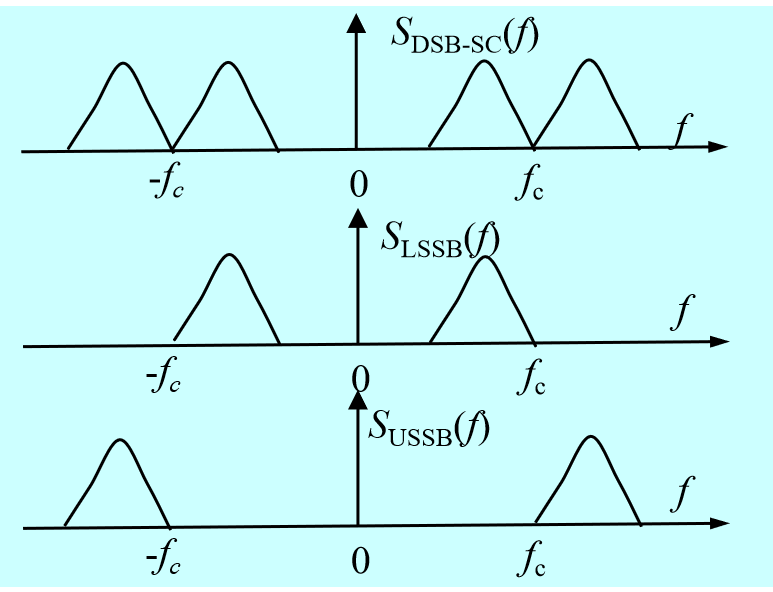图1 双边带信号与下边带以及上边带信号
1、滤波法产生SSB信号
那么如何产生SSB信号呢？从图1中不难看出，我们可以采用滤波的方法，从双边带信号中将下边带以及上边带信号分离出来。图(2)中(a)为SSB调制器，其中$h_{\rm SSB}(t)$可以为下边带滤波器（图b）或者上边带滤波器（图c)，因此单边带信号的波形表达式为
$\tag{1} s_{\rm SSB}(t)=A_cm(t)\cos 2\pi f_ct*h_{\rm SSB}(t),$其傅立叶变换为
$\tag{2} S_{\rm SSB}(f)=\frac{A_c}{2}[M(f-f_c)+M(f+f_c)]H_{\rm SSB}(f).$图3为用滤波法产生下边带（图a）以及上边带（图b）信号示意图。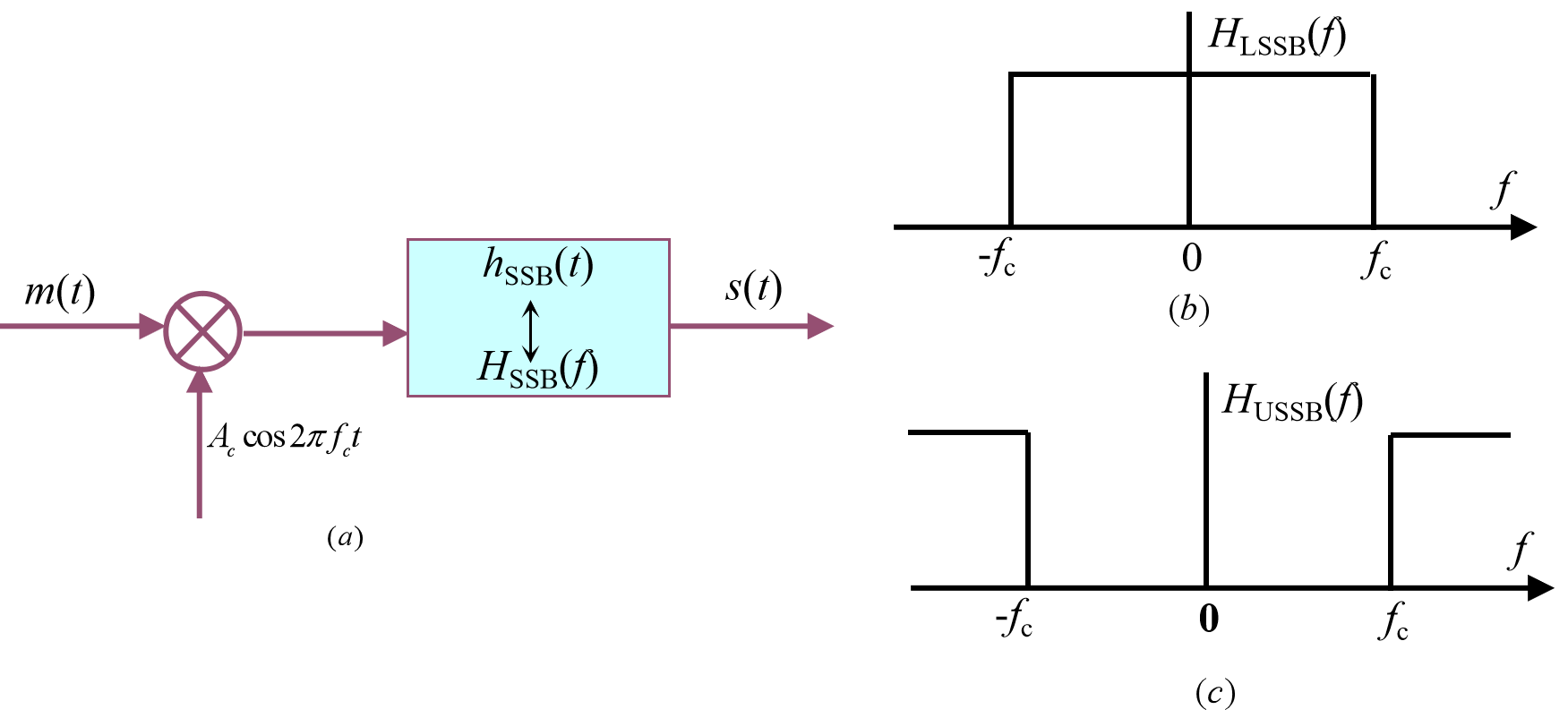图2 SSB调制器模型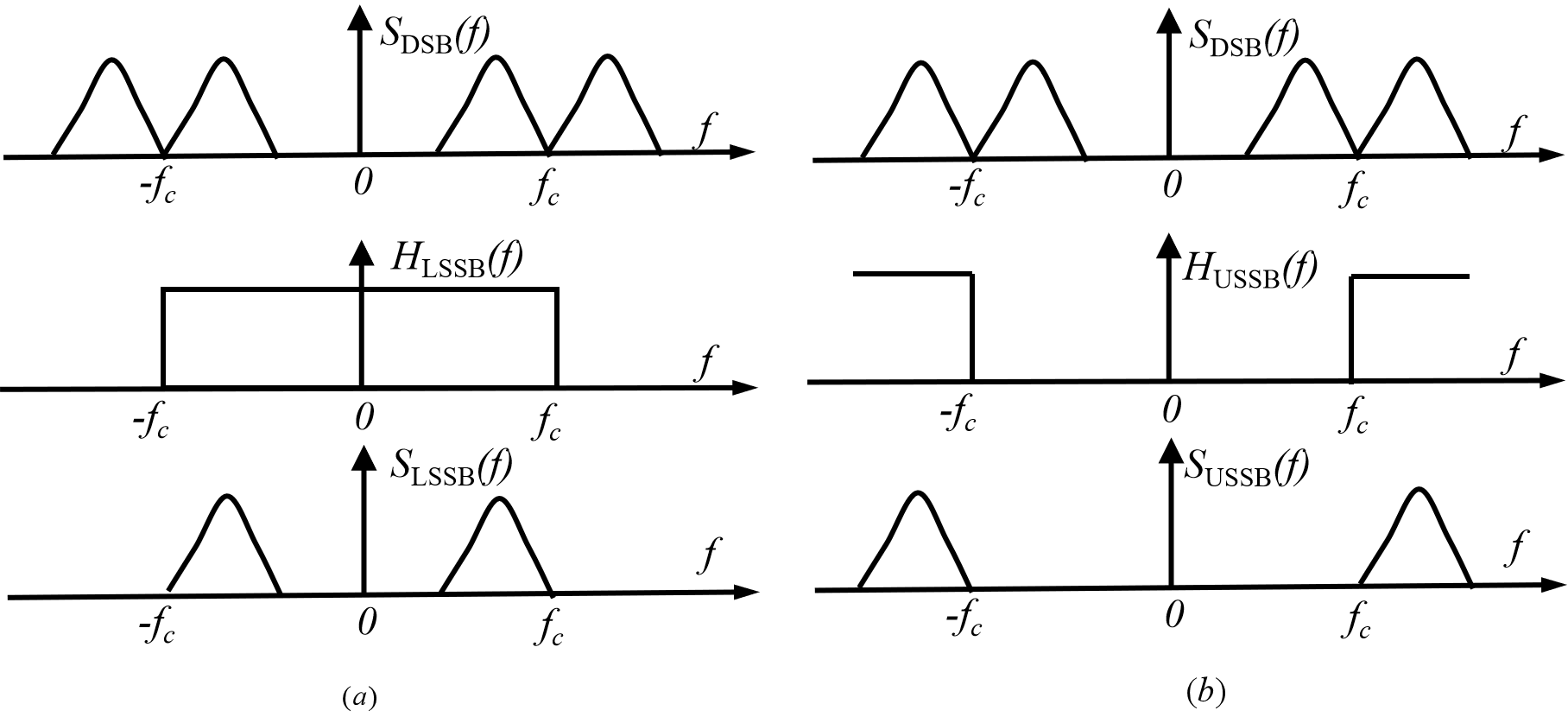图3 滤波器产生单边带信号示意图
2、相移法产生SSB信号
显然，滤波法是从信号的频谱特性出发，从双边带信号中去掉上或者下边带频率成分，从而产生单边信号。那么是否还有其它方法可以产生单边带信号呢？
我们先来看下边带信号，图2(b)中的下边带滤波器可以表示为
$\tag{3} H_{\rm LSSB}=\frac{1}{2}[{\rm sgn}(f+f_c)-{\rm sgn}(f-f_c)],$带入(2)得到下边带信号频谱密度为
\tag{4}\begin{aligned} S_{\rm LSSB}(f)&=\frac{A_c}{2}[M(f-f_c)+M(f+f_c)]H_{\rm LSSB}(f)\\ &=\frac{A_c}{4}[M(f-f_c)+M(f+f_c)]+\frac{A_c}{4}[M(f+f_c)\cdot {\rm sgn}(f+f_c)-M(f-f_c)\cdot {\rm sgn}(f-f_c)]. \end{aligned}我们可以求得(4)的傅立叶反变换为
$\tag{5} s_{\rm LSSB}(t)=\frac{A_c}{2}[m(t)\cos 2\pi f_ct+\hat m(t)\sin 2\pi f_ct].$同理，我们可以推得上边带信号时域表达式为
$\tag{6} s_{\rm USSB}(t)=\frac{A_c}{2}[m(t)\cos 2\pi f_ct-\hat m(t)\sin 2\pi f_ct].$下边带信号的傅立叶变换如图4所示。从图中不难看出，$m(t)\sin 2\pi f_ct$是将上边带频率部分反相，与双边带信号叠加后，上边带部分被正负抵消，因而被消除掉，只保留下边带部分。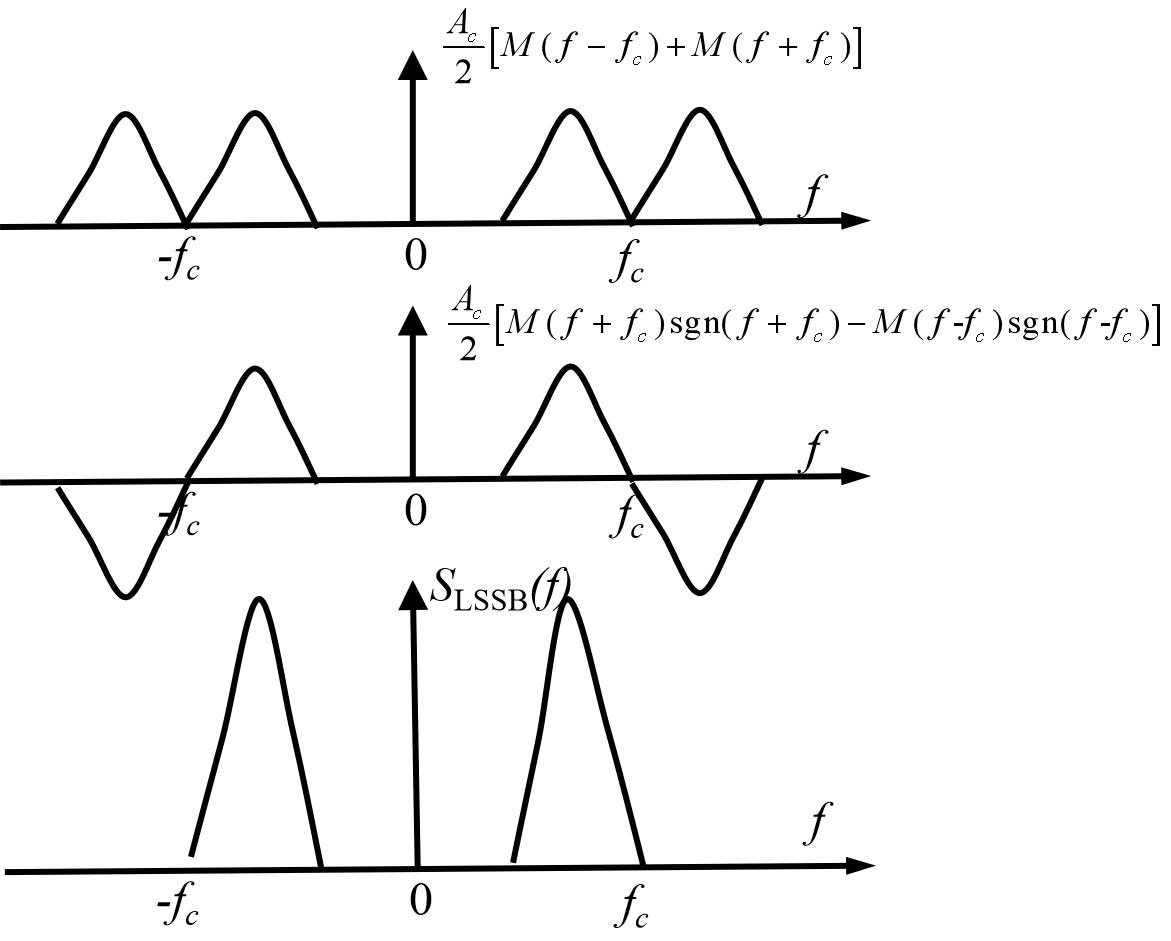图4 相移法产生下边带信号频谱示意图
根据(5)和(6)，我们可以画出相移法模型如图5所示。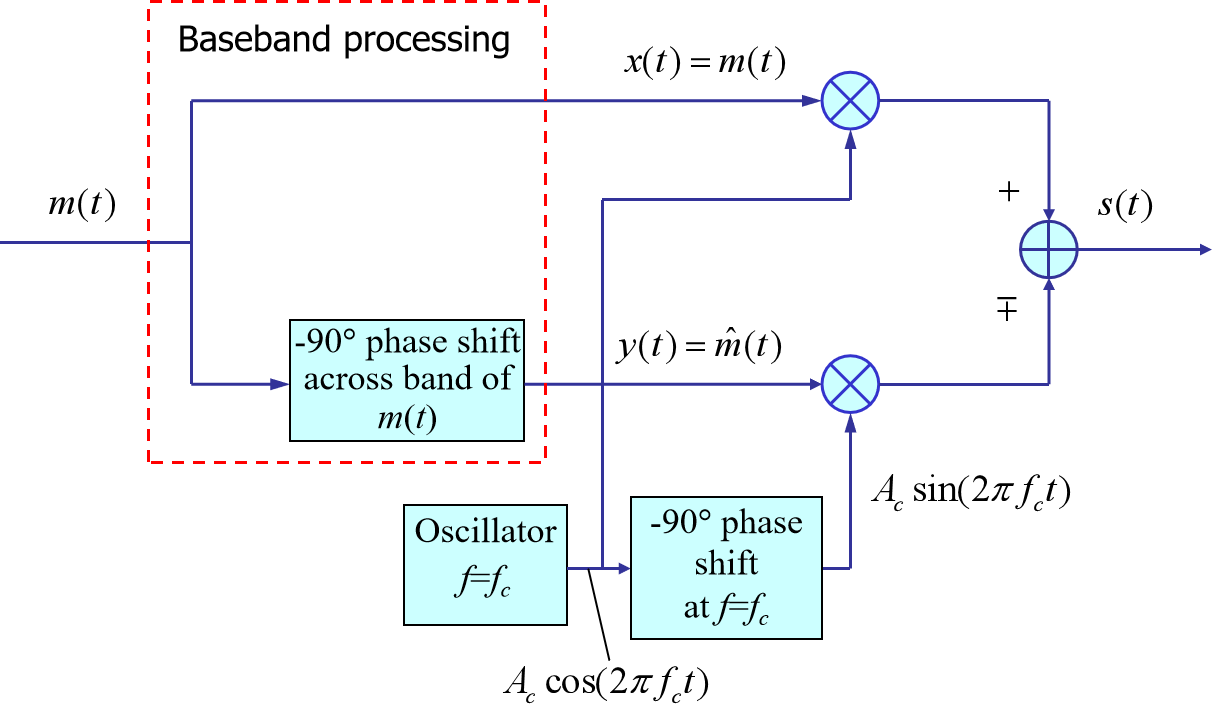图5 相移法产生单边带信号模型


展开全文• 一、MQAM概念 正交振幅调制（QAM）是用两个独立的基带数字信号对两个互相正交的同频载波进行抑制在播的双边带调制，利用这种已调信号在同一带宽内频谱正交的性质来实现两路并行的数字信息传输。 它是把MASK与MPSK...
• AM(Amplitude Modulation)2.3.1、AM-调幅2.3.2、AM-包络检波器2.3.3、调幅系数（调幅度）m2.3.4、AM调制效率（功率利用率）2.4、双边带调制（DSB-SC）2.4.1、双边带调制（DSB-SC）2.4.2、DSB相干解调2.5、单边带调制...
• 幅度调制又可分为常规双边带调制（AM），抑制载波的双边带调制(DSB),单边带调制（SSB）,残留边带调幅等，本文研究信号的单边带调制。在理解单边带原理的基础上，利用滤波法和移相法完成了精度较高的调制。 滤波法：...
• 幅度调制原理1.1 AM调制1.2 双边带调制（DSB）1.3 单边带调制（SSB）1.4 残留边带调制1.5 相干解调与包络检波2 线性调制的抗噪声性能2.1 分析模型2.2 DSB调制系统的性能2.3 SSB调制系统的性能2.4 AM包络检波的性能3...
• 一、调制原理 常规双边带调幅又叫标准调幅，简称调幅（AM）。假设调制信号 m(t) 的平均值为 0，将其加上一个直流分量 A0 后与载波相乘就可以得到AM信号。 调制模型如下图所示： 二、解调原理 对于AM信号来说，使用两...matlab 信号处理
• - DSB信号调制原理 对于DSB调制，来源于AM信号调制，在分析AM信号的频谱结构的时候，其包含载频分量，上边分量和镜像的下边分量，对于AM幅度调制，是将原来的调制信号的频率搬移至了载频所在的更高的点频率位置...
• 目录一、为什么要调制二、幅度调制1、AM调制2、抑制载波的双边带调制（DSB）3、单边带（SSB）4、残留边带（VSB）5、性能分析三、Matlab实现1、调制2、非相干解调3、相干解调四、FPGA实现1、AM信号非相干解调2、DSB...fpga 信号处理 数字信号处理
• 在这里我将用到的是滤波器法，其原理：产生SSB信号的最直观的方法是让双边带信号通过一个边带滤波起保留所需要的一个边带，滤除不要的边带，这只要让它通过一个低通滤波器，而这是很难实现，为此在工程中往往采用...
• 本设计主要涉及模拟电子线路中的放大器工作原理、通信电子线路中高频功率放大器的工作原理，以及对振幅的普通调制和双边带调制原理。对电路基础、模拟电子线路、通信电子线路中的一些基础知识要求较高，是对以往学过...
•          如果在AM调制模型中将直流A0去掉，即可得到一种高调制效率的调制方式——抑制载波双边带信号（DSB—SC），简称双边带调制信号（DSB）。其时域表达式为 sDSB(t)=m(t)coswct s_{DSB}(t) = m(t)cosw...
• 4 单边带调制（SSB）原理及仿真 12 4.1 单边带信号的频域表达 12 4.2 单边带信号的解调 13 4.3 单边带幅度调制（SSB）Simulink仿真 15 5 角度调制 16 5.1 角度调制的涵义 16 5.2 角度调制波形图及表达式 16 5.3 角度...
• 实际上QPSK信号是两路正交双边带信号。现在人们对通信的要求越来越高，高速率、大容量、以及多业务，这些对有限的频谱资源构成了大的挑战。因此，对相移键控的研究具有重要意义，因为信道条件的限制，大多数数字通信...
• 模拟调制系统 3.1 引言 3.2 线性调制的原理 3.3 线性调制系统的解调 3.4 线性调制系统的抗噪声性能分析 3.5 非线性调制系统的原理及抗噪声性能 3.6 各种模拟调制系统的比较 3.1 引言 3.2.2 双边带调制(DSB) ...
• （1）具有离散大载波的双边带调幅又称标准调幅，正弦型载波的幅度将随基带信号成线性变化。 （2）AM信号的频谱与基带信号的频谱呈线性关系，只是将基带信号的频谱从零频线性搬移到±f_c处。如果已调信号的频谱和基带...matlab
• 【 1. 调制与解调 】 调制调制信号去控制载波信号的某一个参量的过程。 解调 ...调制的逆过程，即从已调波中恢复原调制信号。...【 2. 振幅调制AM 】 ...AM信号的生成原理框图 ...【 3....双边带...
• 实际上QPSK信号是两路正交双边带信号。现在人们对通信的要求越来越高，高速率、大容量、以及多业务，这些对有限的频谱资源构成了大的挑战。因此，对相移键控的研究具有重要意义，因为信道条件的限制，大多数数字通信...
• DSB （抑制载波双边带原理笔者不过多赘述，通信原理教材都有详细介绍，直接上干货 %AM调制信号的MATLAB实现 dt=0.001; %时间采样频谱 Fs=100; fm=1; %信源的最高频率 fc=10; %载波中心频率 T=4; %信号时长 N=T/dt...matlab
• ## I/Q 解调原理

千次阅读 2018-12-24 23:12:28
IQ 解调器的工作原理可通过将其 RF 输入信号 sRF(t) 表示为两个双边带调制正交载波的组合来说明：  (1)     如图 A 所示，同相分量 I(t) 和正交分量 Q(t) 为基带信号，可被视作一个产生 sRF(t) ...
• 现介绍一种简易的振幅调制电路，该电路的载波由高频信号发生器产生，经放大后和调制信号经乘法器后，输出抑制载波的双边带调幅波，输出的双边带调辐波与放大后的载波再经过相加器后，即可产生普通调幅波，本电路的...
• DSB调幅原理 用待传输的低频信号去改变高频信号...为了提高信息传输效率，采用双边带振幅调制，其调幅波的表达式为： 式中m=UΩm/Ucmm = U_{Ωm} /U_{cm}m=UΩm​/Ucm​称为调幅系数 波形如图 频谱如图 MC1496电路
• 　根据通信理论，单边带系统将功率全部转换到了有用信息的边带，使用的带宽只有双边带调制信号的一半，具有100%理论传输效率和2倍的频带利用率，成为一种广泛使用的方式.目前，通信设备大多采用单边带系统，信号处理...